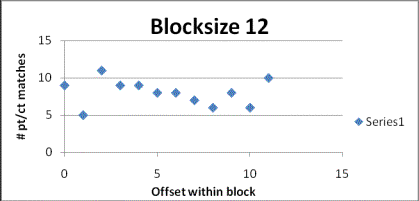# The Chaocipher Challenge: Progress Report #3

Moshe Rubin (mosher@mountainvistasoft.com)
Last updated: 2 March 2009

## Hypothesis

I would like to present evidence that the Chaocipher algorithm requires a 13- or 26-letter blocking.  This supports Greg Mellen's hypothesis that such a blocking was used.

## A case for a 13- or 26-letter blocking factor

In Greg Mellen's seminal article  he hypothesized that the Chaocipher device works on blocks of 13 letters at a time.  He based this on the fact that he believed a pt/ct identity never occurred within a 13-letter block of text.  For example, if the Chaocipher text in Exhibit 1 is divided into blocks of 13 letters, starting with the first letter of the exhibit, we would have the following:
`A L L G O O D Q Q U I C KC L Y T Z P N Z K L D D Q`
`B R O W N F O X E S J U MG F B O O T Y S N E P U A`
`P O V E R L A Z Y D O G TG K I U N K N C R I N R C`
`O S A V E T H E I R P A RV K J N H T O A F Q P D Petc. . . .`
The above division assumes a block begins with the first letter of the text ("offset 0").  If we assume the blocking begins with an offset of 1 we would get the follows:

`                        A                        C`
`L L G O O D Q Q U I C K BL Y T Z P N Z K L D D Q G`
`R O W N F O X E S J U M PF B O O T Y S N E P U A G`
`O V E R L A Z Y D O G T OK I U N K N C R I N R C V`
`S A V E T H E I R P A R TK J N H T O A F Q P D P N`
Continuing in this fashion, there are in total 13 ways to divide the text into blocks of 13 letters.

Similarly, we can divide the text into blocks of 10, 11, 12, 14, 15, etc. letters.

I wrote a Perl script that counted the number of pt/ct identities in each block, for every block size and offset.  The raw results can be seen here.  For example, blocking size 13 shows the following:

 Block size Offset Number Pt/Ct Identities First identity offset 13 0 8 6253 1 11 389 2 13 389 3 11 3689 4 13 3480 5 12 1347 6 14 3205 7 13 994 8 12 994 9 7 5868 10 9 1940 11 6 1445 12 5 8893

The results shows that 13-letter blocking with an offset of 0 resulted in 8 pt/ct identities, while an offset of 1 had 11 pt/ct identities.  The last column, for reference, denotes the offset within the text where the first pt/ct pair of the first identity occurred.

## What happens when we graph the block size/offset results?

At this point I graphed each block size N, mapping all N offset results.  For example, mapping block size 12 gives the following graph:The x-axis denotes the offset within a block size of 12 (i.e., 0, 1, 2, ... 11), while the y-axis shows the number of pt/ct identities for that offset.  For this graph we see multiple nodes with possible skewing to the right.

I produced graphs for block sizes 12-14, 22-28, 39, and 52.  You can see all the graphs here.  The results are amazing: block sizes 13 and 26 are clearly normally distributed while the others are clearly not.  Block sizes 39 and 52 (i.e., multiples of 13/26) do not seem to show normal distribution.  Block sizes 13 and 26 are obviously causal while other block sizes introduce random results.

My explanation is:
• The underlying algorithm has uses a blocking of 13 letters (or possibly a 26-letter block size)
• An offset of  0 results in the smallest number of pt/ct identities.
• Previous progress reports have shown that Chaocipher tends to produce a small number of pt/ct identities.
• As the offset grows adjacent blocks begin to overlap and straddle more and more.  This results in a dulling of the small expected number of pt/ct identities (i.e., an increase in non-causal identities).

## Conclusion

• The underlying algorithm indeed incorporates a 13-letter blocking step.
• It could be argued that there blocksize is 26 and that the block size 13 graph is due to its being a factor of 26.
• Future hypotheses should consider this important finding.

# References

 Mellen, Greg.  1979.  J. F. Byrne and the Chaocipher, Work in Progress.  Cryptologia, 3(3): 136-154.